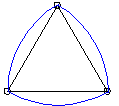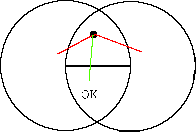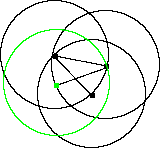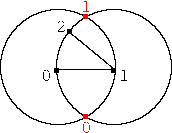# Curves of constant width

The purpose of this page is to describe curves of constant width.

## Definitions

### What is a curve of constant width?

Take two parallel tangents to a curve C. The width of the curve with respect to these tangents is then the distance between these two lines. The diameter of C is the largest width for the curve.
A curve is said to have constant width if the width is identical no matter which direction the supporting tangents are in. In a curve of constant width, it is clear that the (constant) width must be equal to the diameter. This fact is important in several of the proofs.
For example, a circle is the simplest curve of constant width. It has a constant width (r), which is identical to its diameter. Another basic curve of constant width, the Reuleaux Triangle (RT) will be further discussed later.The Reuleaux triangle

### Pinching sets

When talking about construction of a curve of constant width, it is useful to define a pinching set. Just by looking at the picture of the RT, it can been seen that it seems to have a basis of an equilateral triangle (this is where the triangle part comes from.
Formally, a pinching set V is a set of points on the boundary of a curve C of constant width such that every diameter of C is incident with at least one point on V. In the case of the RT above, the vertices of the triangle are the pinching set. A circle has a pinching set of infinite size.

### Reuleaux Polygons

A Reuleaux polygon (or RP) is a curve of constant width which admits a finite pinching set. (This definition excludes the circle, which has an infinite pinching set). The number of vertices in the pinching set is referred to as the order of the RP. As will be shown, all RPs have an odd order, and for any odd integer n > 3, there exists an RP of order n. The set of vertices making up an RP is referred to as a VRP.A RP of order 5

## Motivation

The goal here is to produce any of the infinite number of RPs in linear time. Producing a single RP of degree n is trivially possible by taking a regular star-shaped polygon with n vertices and joining them as described below. This does not, however, give us an arbitrary RP. In order to do this, we need to keep track of the limitations, to ensure that we end up with a valid polygon of constant width. An algorithm to do so is described here.

## Proofs

### All the edges of a VRP have the same length

Let the distance between the first two vertices be (for simplicity) 1. The width of the shape along that line is then 1. By the definition of the VRP, all vertices are on the convex boundary of the RP.
Now, assume that there exists an edge of length > 1. Then we could take two tangents perpendicular to that edge, and the width between those tangents would be > 1.
Similarly, if there were an edge with length < 1, we could get a width of < 1.
This would mean that we have a width != 1, as well as a width = 1, and so the curve would not be of constant width. Thus no edge in the VRP can be greater than 1, and no edge can be smaller than 1, and so all edges in the VRP must have distance equal to 1.

### All vertices of a VRP have distance <= 1

Assume that there exists two vertices whose distance is > 1. Then pick the tangents to the line joining those two vertices, and consider that width. It will be > 1, and so the curve will not have constant width. From above, adjacent vertices have distance 1, and so the non-adjacent vertices will have distance < 1. Note that since VRP is a pinching set, all the vertices must lie on the boundary.

### Each new edge touches all other existing edges

Consider a VRP of order n. Firstly, it is clear that n must be at least 3. I have already shown the RT, which has order 3, so we can consider n > 3.
Now consider the edge between v1 and v2. From above, this has length 1. Now add the edge between v2 and v3. Again, this has distance 1, and the distance between v1 and v3 is less than 1. Each additional vertex must lie within the circle of radius 1 about v1, as well, or there would be two points (v1 and vi) with distance > 1, which is not permitted, as shown above. Similarly, each vertex must lie within the circle of radius 1 from all other points.
This implies that each edge must touch all other edges. The reason for this is that if it did not, then the new vertex would be too far from one of the vertices of the untouched edge.Any edge of the VRP must cross the existing edge

### The order of a RP is odd

From above, each additional edge (with length 1) must cross all the existing edges, including |v1,v2|. This implies that odd vertices are on one side of this edge, and even vertices are on the other.
However, the edge between vn and v1 has length 1 (by construction), and since |vn-v3| < 1, vn must be on the same side as v3. So vn must be odd, and thus a VRP has an odd number of vertices.

### Any valid VRP forms an RP

If we have a VRP, then we can obtain an RP as follows:
For each vertex vi in the VRP, draw an arc (of radius 1) with center vi between its two adjacent points.

The above two proofs, when combined, prove that there exists a VRP of order n for all odd n >= 3.

## Constructing a VRP

In order to construct a RP, we need the set of vertices in the RP. Once we have the VRP, constructing the RP can be done in linear time, using the construction proof given above.

### Finding a VRP in quadratic time

The above discussion suggests a simple construction of the VRP. The idea behind this is to place points one by one into an area satisfying the requirements. We are trying to find an RP of order n.

1. Choose v1 in R2 and v2 as any point at distance 1 from v1.
2. If n=3, then we can pick either point at distance 1 from both v1 and v2, and then stop.
3. Else, for each v3 to vn-1:
4. Choose vx from the intersection of the disks of radius 1 centered at vi for all i from 1 to x-2, intersected with the disk of radius 1, centered at vx-1The next vertex must lie on the boundary of the green circle, and within all the other circles.

5. Then the last point, vn, is simply the point at distance 1 from both v1 and vn-1. As n is odd, we pick the point on the correct side (which is unique)

It is clear that this solution satisfies the constraints (by construction). This solution takes quadratic time. However, we also need to prove that such a point will always exist. This follows from the proofs above. At each stage, the algorithm adds an additional circle to the requirements, and we need to prove that the intersection of this circle with the preceeding circles is not the empty set. This can be seen from the above diagram - each added circle must at least contain the previous point, as well as the line itsself. These were, by defintion, part of the original intersection, and they are part of the new circle, so they are part of the combined intersection. Thus the combined area is not empty, and so the algorithm works.

### A linear time solution

The above solution is quadratic because every added point must examine all preceding points. This is clearly inefficient. An obvious improvement would be to avoid requiring this additional lookup. So, how do we do this?

At each step, we can see that we add additional restrictions to the required area. However, the final point lies on an arc, center the previous point, and bounded by the boundaries of other circles. The linear time algorithm produces, at each stage, the points bounding this arc.

This is done by keeping track of the restricted area as follows. Note that the points are numbered 0 to n-1.

1. Choose v0 in R2 and v1 as any point at distance 1 from v0.
2. If n=3, then we can pick either point at distance 1 from both v0 and v1, and then stop.
3. else, choose v2 anywhere at distance 1 from v1 (apart from v0)
4. Then we consider the intersections of the two circles of radius 1 centered at v0 and v1. There are two such points - label the one on the v2 side as p1 and the other asp0.
5. Now, for each other point, we update pm for even m such that we form a bound on the arc, by setting it to the intersection of the previous points' circle and the arc of radius 1 centered at v0 and bounded by vi and pm-2.
6. For odd m we do the same, except the arc is centered at v1, and bounded by v0 instead. This is because of the alternating nature of the points, as discussed before.
7. The last point behaves as in the quadratic algorithm, choosing the single remaining position.

This algorithm takes a linear amount of time, since each point is only examined once.

Heres an example showing a few steps of the algorithm (black points are part of the VRP; red points are p)3 points 4 points 5 points

## The applet

There are two applets. The first one was written by myself from scratch. Given an odd n > 3, it generates a random RP of order n. It implements the quadratic algorithm. Note that this sometimes locks up under Netscape. It never locks up under the applet viewer, so I have been unable to debug this - it may be a browser issue. There is also a small amount of numerical instability in the algorithm, and so occasionally the final point will have distance > 1, so the arc will be invalid.
To use the applet, type an odd integer > 3 into the text field, and press enter.

source code

The other applet shows the idea behind the linear time solution. You can start with a regular star-shaped polygon, and distort it, dragging each point along what would be the limited arc. You can also see what happens to the resulting RP when all of the edges do not cross. It was not written by me, and source is not available. To see this applet, click here. (This is an external site which discourages downloading the applet directly)

## Bibliography

1
A linear time Construction of Reuleaux Polygons, Yaakov Kupitz, Horst Martini, Bernd Wegner, Beitrage zur Algebra und Geometrie, Contributions to Algebra and Geometry, Volume 37 (1996), No. 2, 415-527.

2
Curves of Constant Width, http://www.cut-the-knot.com/do_you_know/cwidth.html and http://www.cut-the-knot.com/Curriculum/Geometry/CWStar.html